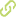# Thermal performance of a parabolic trough linear collector using Al2O3/H2O nanofluids Academic Article in Scopus•
• Overview
•
• Identity
•
•
• View All
•

### abstract

• © 2018 Elsevier Ltd The effect of using nanofluids on the thermal performance of a parabolic trough linear collector (PTLC) was investigated. Experiments were performed to evaluate the thermal efficiency of a PTLC according to the ISO 9806 test. Distilled and deionized water and nanofluids produced by dispersing nanoparticles of alumina (Al 2 O 3 ) in water with a volume fraction of 1% and 3% were employed as a heat transfer fluid (HTF). Adding nanoparticles increased the thermophysical properties of the base fluid, enhancing the heat transfer between the receiver tube and the working fluid. An increase in the thermal efficiency of the PTLC with respect to the base fluid was achieved for all the nanofluids tested in different ambient conditions and incident angles. For the first set of experiments, maximum efficiencies observed were: 52.4% for 3% volume fraction nanofluid and 40.8% for water. For experiments run later in the year, maximum efficiencies obtained were 57.7% for nanofluid with 1% volume fraction and 46.5% for water. Also, it was found that the efficiency of the nanofluids had a strong dependence on the incident angle, where the highest efficiency was obtained for the smallest angle.
• © 2018 Elsevier Ltd The effect of using nanofluids on the thermal performance of a parabolic trough linear collector (PTLC) was investigated. Experiments were performed to evaluate the thermal efficiency of a PTLC according to the ISO 9806 test. Distilled and deionized water and nanofluids produced by dispersing nanoparticles of alumina (Al2O3) in water with a volume fraction of 1% and 3% were employed as a heat transfer fluid (HTF). Adding nanoparticles increased the thermophysical properties of the base fluid, enhancing the heat transfer between the receiver tube and the working fluid. An increase in the thermal efficiency of the PTLC with respect to the base fluid was achieved for all the nanofluids tested in different ambient conditions and incident angles. For the first set of experiments, maximum efficiencies observed were: 52.4% for 3% volume fraction nanofluid and 40.8% for water. For experiments run later in the year, maximum efficiencies obtained were 57.7% for nanofluid with 1% volume fraction and 46.5% for water. Also, it was found that the efficiency of the nanofluids had a strong dependence on the incident angle, where the highest efficiency was obtained for the smallest angle.

• July 1, 2018
• July 1, 2018